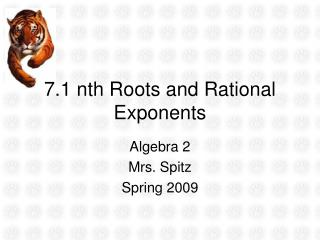Download Presentation7.1 nth Roots and Rational Exponents

# 7.1 nth Roots and Rational Exponents

Download Presentation## 7.1 nth Roots and Rational Exponents

- - - - - - - - - - - - - - - - - - - - - - - - - - - E N D - - - - - - - - - - - - - - - - - - - - - - - - - - -
##### Presentation Transcript

1. 7.1 nth Roots and Rational Exponents Algebra 2 Mrs. Spitz Spring 2009

2. Objectives/Assignment • Evaluate nth roots of real numbers using both radical notation and rational exponent notation. • Use nth roots to solve real-life problems such as finding the total mass of a spacecraft that can be sent to Mars. Assignment: pp. 404-405 #4-61 every 3rd

3. Evaluating Nth Roots • You can extend the concept of a square root to other types of roots. For instance 2 is a cube root of 8 because 23 = 8, and 3 is a fourth root of 81 because 34 = 81. In general, for an integer n greater than 1, if bn = a, then b is an nth root of a. An nth root of a is written , where n is the index of the radical.

4. Evaluating Nth Roots • You can also write an nth root of a as a power of a. For the particular case of a square root, suppose that . Then you can determine a value for k as follows: Definition of a square root Substitute ak for Product of powers property Set exponents equal when bases are equal. Solve for k

5. Evaluating Nth Roots • Therefore, you can see that . In a similar way, you can show that and . In general, for any integer n greater than 1

6. Ex. 1 Finding nth Roots • Find the indicated real nth root(s) of a. A. n = 3, a = -125 Solution: Because n = 3 is odd, a = -125 has one real cube root. Because (-5)3 = -125, you can write: or

7. Ex. 1 Finding nth Roots • Find the indicated real nth root(s) of a. B. n = 4, a = 16 Solution: Because n = 4 is even, a = 16 > 0, has two real fourth roots. Because 24 = 16, and (-2)4 = 16, you can write: or

8. A rational exponent does not have to be of the form where n is an integer greater than 1. Other rational numbers such as and can also be used as exponents.

9. Ex. 2 Evaluating Expressions with Rational Exponents A. B. Using radical notation Using rational exponent notation. OR OR

10. Study Tip • When using a graphing calculator to approximate an nth root, you may have to rewrite the nth root using a rational exponent. Then use the calculator’s power key.

11. Ex. 3 Approximating a Root with a Calculator • Use a graphing calculator to approximate: SOLUTION: First rewrite as . Then enter the following: To solve simple equations involving xn, isolate the power and then take the nth root of each side.

12. A. 2x4 = 162 B. (x – 2)3 = 10 Ex. 4 Solving Equations Using nth Roots

13. Ex. 5: Using nth Roots in Real Life • The total mass M (in kilograms) of a spacecraft that can be propelled by a magnetic sail is, in theory, given by: where m is the mass (in kilograms) of the magnetic sail, f is the drag force (in newtons) of the spacecraft, and d is the distance (in astronomical units) to the sun. Find the total mass of a spacecraft that can be sent to Mars using m = 5,000 kg, f = 4.52 N, and d = 1.52 AU.

14. Solution The spacecraft can have a total mass of about 47,500 kilograms. (For comparison, the liftoff weight for a space shuttle is usually about 2,040,000 kilograms.

15. Ex. 6: Solving an Equation Using an nth Root • NAUTICAL SCIENCE. The Olympias is a reconstruction of a trireme, a type of Greek galley ship used over 2,000 years ago. The power P (in kilowatts) needed to propel the Olympias at a desired speed, s (in knots) can be modeled by this equation: P = 0.0289s3 A volunteer crew of the Olympias was able to generate a maximum power of about 10.5 kilowatts. What was their greatest speed?

16. SOLUTION • The greatest speed attained by the Olympias was approximately 7 knots (about 8 miles per hour).